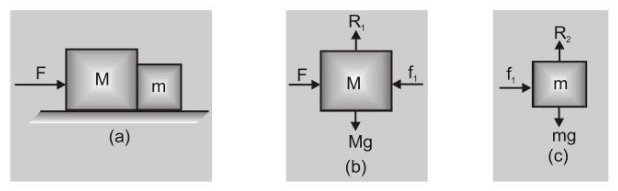Download the eSaral app and start learning from Kota's top IITians and doctors.

# What is Free Body Diagram?## Free Body Diagram

A diagram showing all external forces acting on an object is called "Free Body Diagram" F.B.D.

In a specific problem, first, we are required to choose a body and then we find the number of forces acting on it, and all the forces are drawn on the body, considering it as a point mass. The resulting diagram is known as the free body diagram (FBD).

For example, if two bodies of masses $m$ and $M$ are in contact and a force $F$ on $M$ is applied from the left fig. (a), the free body diagrams of $M$ and $m$ will be as shown in fig. (b) and (c).Important Point :

Two forces in Newton's third law never occur in the same free-body diagram. This is because a free-body diagram shows forces acting on a single object, and the action-reaction pair in Newton's third law always act on different objects.